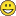# New to Qlik Sense

Discussion board where members can get started with Qlik Sense.

Highlighted
Contributor

## Expression Possibility

Hi guys,

Can you do something in Qlik via a function/expression to determine if a character in a field is either a text or a number.

I have some postcode data like

• LA2
• L40

I want to be able to say if the first character is a letter and the second is a number then do something.  If the first character is a letter and the second character is also a letter then do something else..

Is there a function to do this by any chance?

Thanks

J

Tags (3)
1 Solution

Accepted Solutions
Contributor II

## Re: Expression Possibility

Hi Jamel!

Try this inside Qlikview:

IF(

ISTEXT(LEFT(CODE, 1)) AND ISNUM(RIGHT(LEFT(CODE, 2),1)),

'action 1',

if(ISTEXT(LEFT(CODE, 1)) AND ISTEXT(RIGHT(LEFT(CODE, 2),1)), 'action2'))Rgds,

Benazir

11 RepliesLuminary

## Re: Expression Possibility

we use applymap to do that and precalculate in the script.

It takes seconds to implement with excel

M:

MAPPING LOAD * INLINE [

From, To

LA, 0

L2, 1

];

...

if(applymap('M',fieldname) = 1, 'Do sth', 'Do sth else')

...

Contributor

## Re: Expression Possibility

Thanks Dion.

I am actually loading this data into Qlik from Excel, so is there a function to do this in Excel? If so, i can sort this from the source!!

Thanks

J

Contributor II

## Re: Expression Possibility

Hi Jamel

If( istext( Left( Postcode, 1)) and isnum( Left( Postcode, 2)), 'Then', 'Else' ) as X

istext and isnum returns true/false

/TeisLuminary

## Re: Expression Possibility

yes, create a lookup table and use vlookup

https://www.timeatlas.com/vlookup-tutorial/Luminary

## Re: Expression Possibility

Is correct, but this means behaviour is the same across all values. In reality this does not always happen.

Thus the suggestion for a mapping table.

Contributor II

## Re: Expression Possibility

Hi Jamel!

Try this inside Qlikview:

IF(

ISTEXT(LEFT(CODE, 1)) AND ISNUM(RIGHT(LEFT(CODE, 2),1)),

'action 1',

if(ISTEXT(LEFT(CODE, 1)) AND ISTEXT(RIGHT(LEFT(CODE, 2),1)), 'action2'))Rgds,

Benazir

Contributor

## Re: Expression Possibility

This worked a treat. Thanks Benazir and thanks everyone else for your assistanceContributor II

## Re: Expression Possibility

Happy to help JamelContributor II

## Re: Expression Possibility

What a steal congrats